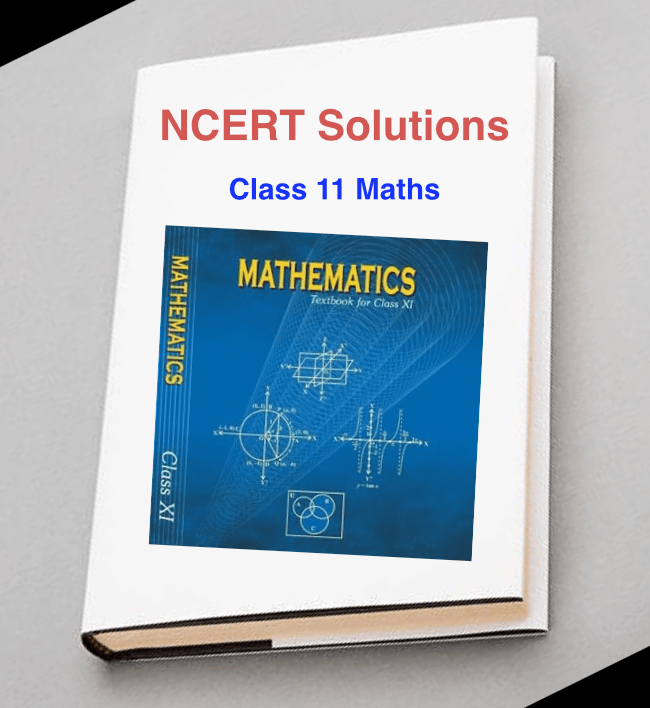# NCERT Solutions For Class 11 Maths English Medium In PDF

NCERT Books are very useful for students who want to score good percentage in their Exams. We provide NCERT textbooks and solution for all class 8, class 9, class 10, class 11, class 12. In this post, We provide NCERT Solutions for Class 11 Maths in PDF format with direct download option.

NCERT Solutions being necessary for those who desire to score well in their class 11 maths examination. The solution covers every question and improve students to prepare in a good manner and score high percentage marks. Now students download the class 11 maths NCERT solutions of all chapters in full detailed manner.For those students who have difficulty in getting solutions to their problem chapter can refer to the NCERT solutions. Students don’t worry about NCERT Solutions for Class 11 Maths, you can download these class notes free of cost from our site.

The NCERT Math solution class 11 are made by our expert and experienced teachers. We strongly suggest students to exercises and practicing these solutions, by this students get maximum marks in their examination.

#### Chapter 1: Sets

Chapter 2: Relations and Functions

Chapter 3: Trigonometric Functions

Chapter 4: Principle of Mathematical Induction

Chapter 5: Complex Numbers and Quadratic Equations

Chapter 6: Linear Inequalities

Chapter 7: Permutations and Combinations

Chapter 8: Binomial Theorem

Chapter 9: Sequences and Series

Chapter 10: Straight Lines

Chapter 11: Conic Sections

Chapter 12: Introduction to Three Dimensional Geometry

Chapter 13: Limits and Derivatives

Chapter 14: Mathematical Reasoning

Chapter 15: Statistics

#### Key points of NCERT Solutions :

• These Solutions given are entirely FREE
• Accurate and qualified answers given for each question in textbooks
• All solution are handmade like Class Notes
• The solution is Created by subject matter expert teachers
• The Class 11 maths NCERT solutions are in pdf format also.
• NCERT Math solution class 11 is chapter wise Solution of each chapter.

Hence, we recommend the students NCERT Solutions for Class 11 Maths reading these solutions thoroughly and making your study very well.

The NCERT Solutions for Class 11 Maths present in-depth and step-by-step solutions to all questions and we support to students that will help them in working on assignments, learning and preparing for the examination.Written by

##### Sonam Sharma

I am govt employ and if you have any question related to govt job then please ping me. Downlaod NCERT Books For Class 10 &NCERT Class 10th Maths Book

This site uses Akismet to reduce spam. Learn how your comment data is processed.